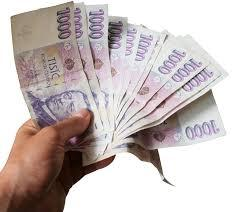# Money 6

Lita had some money. She spent 1/2 of it on a handbag and 1/3 of the remainder on the blouse. She had 260.00. How much money did she have at the start?

x =  1560

### Step-by-step explanation:

x-x/2-x/3 = 260.00

x = 1560

x = 1560/1 = 1560

x = 1560

Our simple equation calculator calculates it.Did you find an error or inaccuracy? Feel free to write us. Thank you!

Tips for related online calculators
Do you have a linear equation or system of equations and looking for its solution? Or do you have a quadratic equation?